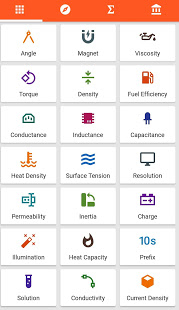174°

eingestellt am 13. JunProduktdetails
✓ Built-In real-time Currency Converter and their latest exchange rates.
# Unit Converter.
# Currency Rate.
# Metric Conversion.
# Conversion.
# Metric Conversion Table.
# Converter.
# 48 unit categories.
# 12800+unit conversions.
# Smart Tools - Bubble Level, Compass, Protractor, Resistor codes, Stop Watch, Ruler, World Time, Date Converter and much more.
# Currency Converter - now supports 162 world currencies with latest exchange rates
# Bit Coin conversion - Latest Bit coin Exchange rates and conversion.
# Has the highest number of unit conversion categories among all other unit converters.
# Now added Financial Calculators and Mathematical Calculators along with other unit converters.
# Time Zone Calculator with day light savings and accurate time differences calculations.
#

Has most important conversion tools that are used in daily life

including Fuel Calculations, Temperature, Volume, Speed, Weight,

Computer Storage, Angle, Power, Viscosity, Force, Energy, Torque,

Density and much more…
# Has Engineering conversion tools like

radiation, electric resistance, electric capacitance, inductance,

inertia, specific heat density, specific heat capacity, and

illumination.
# Built-In Calculator to perform basic arithmetic operations on the fly while using the unit converter.
# Supports both Metric and Imperial Units.
# A Feature to add and customize Favourite Units.
#

Financial calculators - Loan calculator, Compound interest calculator,

Retirement calculator, Service tax calculator, Stock calculator
# Maths calculators -Roman numeral converter, Number base converter, Number series generator, Ratio, Fraction, Proportion, etc..
# First ever Unit Conversion App to feature and support Latest Material design.
Zusätzliche Info

### Gruppen

3 Kommentare
Währungskurse sind fast 1 Jahr alt also völlig nutzlos
Dein Kommentar@
Text